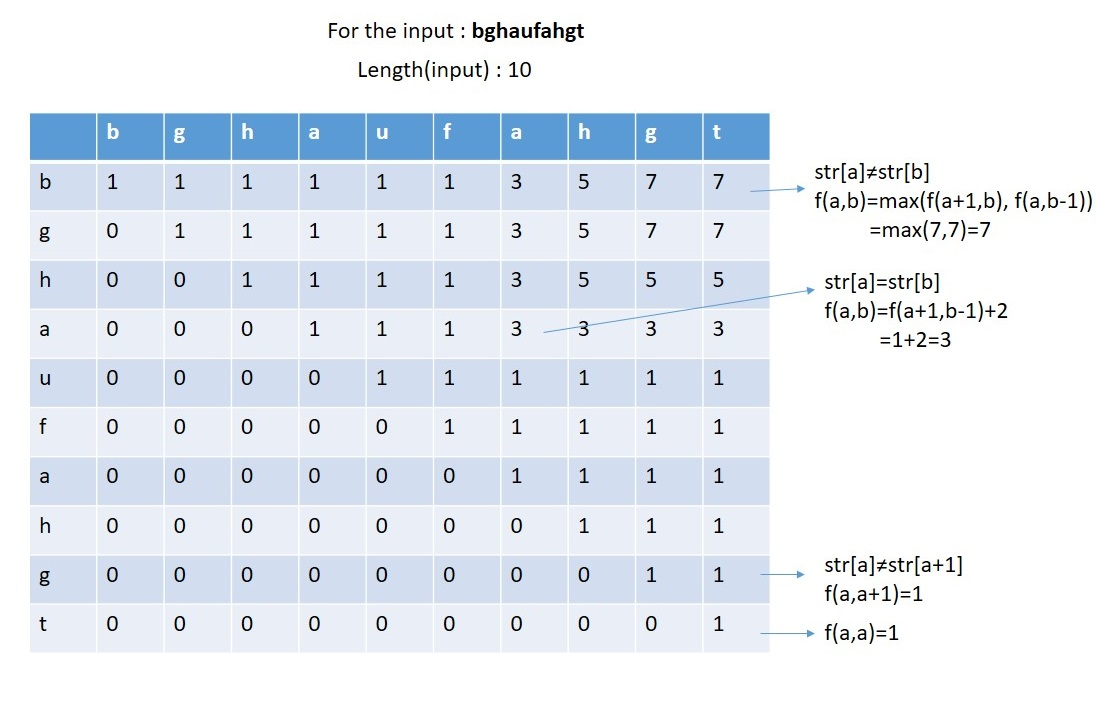Q:

# Find out the length of the longest palindromic subsequence from a string

Given a string you have to find out the length of the longest palindromic subsequence from the given string.

```    Input:
T Test case
T no of input string will be given to you.

E.g.
3

bghaufaght
souabbuos
sahajkhahas

Constrain
1≤ length (string) ≤100

Output:
Print the length of the longest palindromic sub sequences from that string
```

Example

```    T=3

Input:
bghaufahgt

Output:
7 (ghafahg)

Input:
souabbuos

Output:
8 (soubbuos)

Input:
sahajkhahas

Output:
9 (sahajahas)```

Let there is a string str.

Now possible arrangements are:

1. Single middle characters like aba
2. Paired middle characters like bb

Let, f(a,b) = length of palindromic substring from index a to index b.

Considering the above three facts:

1. If there is only one character then f(a,a) = 1
2. If there are two characters a and a+1 both are same then f(a,a+1) = 2 if both are not same then f(a,a+1) = 1.
3. If there is a substring starting from index a to index b then,
1. If str[a] and str[b] both are same then f(a,b) = f(a+1,b-1) + 2
2. If str[a] and str[b] both are not same then f(a,b)= max{ f(a+1,b) , f(a,b-1) }

For, str = bghaufahgtC++ Implementation:

``````#include <bits/stdc++.h>
using namespace std;

int length_of_palindrom(string str)
{
int len = str.length();
int arr[len][len];
memset(arr, 0, sizeof(arr));
for (int i = 0; i < len; i++) {
arr[i][i] = 1;
}
//for the paired middle character
for (int i = 0; i < len - 1; i++) {
if (str[i] == str[i + 1])
arr[i][i + 1] = 2;
else
arr[i][i + 1] = 1;
}
//length wise traversal
for (int l = 3; l <= len; l++) {
for (int i = 0; i <= len - l; i++) {
int j = i + l - 1;
if (str[i] == str[j])
arr[i][j] = arr[i + 1][j - 1] + 2;
else
arr[i][j] = max(arr[i + 1][j], arr[i][j - 1]);
}
}
return arr[len - 1];
}

int main()
{
int t;
cout << "Test Case : ";
cin >> t;
while (t--) {
cout << "Enter the string : ";
string str;
cin >> str;
cout << "Length of the palindrome: " << length_of_palindrom(str) << endl;
}
}
``````

Output

```Test Case : 3
Enter the string : bghaufahgt
Length of the palindrome: 7
Enter the string : souabbuos
Length of the palindrome: 8
Enter the string : sahajkhahas
Length of the palindrome: 9```